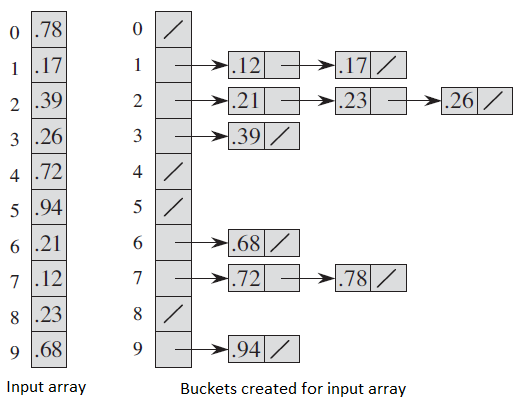# Bucket Sort

Bucket sort is mainly useful when input is uniformly distributed over a range. For example, consider the following problem. Sort a large set of floating point numbers which are in range from 0.0 to 1.0 and are uniformly distributed across the range. How do we sort the numbers efficiently?

A simple way is to apply a comparison based sorting algorithm. The lower bound for Comparison based sorting algorithm (Merge Sort, Heap Sort, Quick-Sort .. etc) is \Omega(nLogn), i.e., they cannot do better than nLogn. Can we sort the array in linear time? Counting sort can not be applied here as we use keys as index in counting sort. Here keys are floating point numbers. The idea is to use bucket sort. Following is bucket algorithm.

bucketSort(arr[], n)
1) Create n empty buckets (Or lists).
2) Do following for every array element arr[i].
.......a) Insert arr[i] into bucket[n*array[i]]
3) Sort individual buckets using insertion sort.
4) Concatenate all sorted buckets.

Following diagram (taken from CLRS book) demonstrates working of bucket sort.// C++ program to sort an array using bucket sort// C++ program to sort an array using bucket sort #include
#include <algorithm>
#include <vector>
using namespace std;
#include <iostream>
#include <algorithm>
#include <vector>
using namespace std;

// Function to sort arr[] of size n using bucket sort
void bucketSort(float arr[], int n)
{
// 1) Create n empty buckets
vector <float> b[n];

// 2) Put array elements in different buckets
for (int i=0; i<n; i++)
{
int bi = n*arr[i]; // Index in bucket
b[bi].push_back(arr[i]);
}

// 3) Sort individual buckets
for (int i=0; i<n; i++)
sort(b[i].begin(), b[i].end());

// 4) Concatenate all buckets into arr[]
int index = 0;
for (int i = 0; i < n; i++)
for (int j = 0; j < b[i].size(); j++)
arr[index++] = b[i][j];
}

/* Driver program to test above funtion */
int main()
{
float arr[] = {0.897, 0.565, 0.656, 0.1234, 0.665, 0.3434};
int n = sizeof(arr)/sizeof(arr);
bucketSort(arr, n);

cout << "Sorted array is \n";
for (int i=0; i<n; i++)
cout << arr[i] << " ";
return 0;
}

Output:
Sorted array is
0.1234    0.3434    0.565    0.656    0.665    0.897

Hi I am Alfred.